# TensorFlow2.1入门学习笔记(2)——Matplotlib数据可视化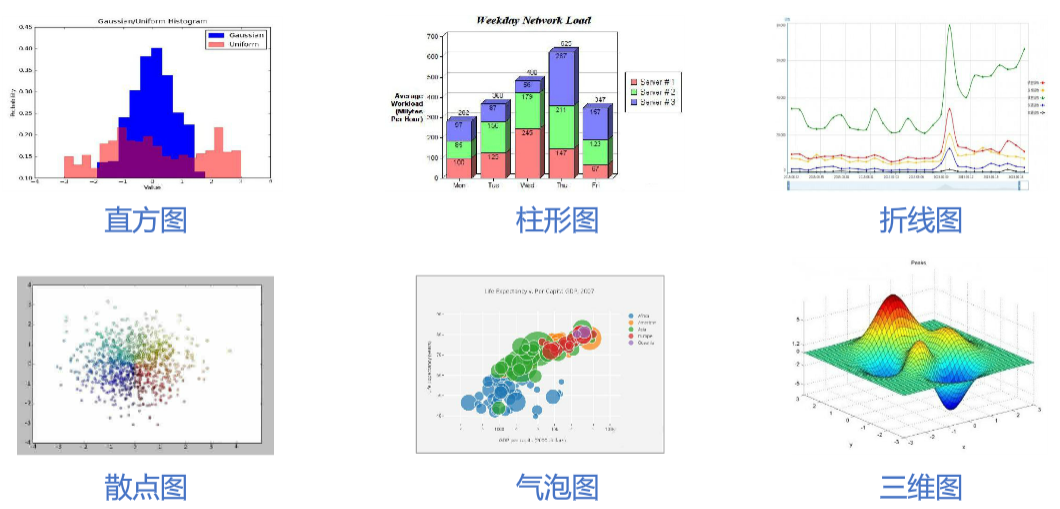#### 安装Matplotlib库

 1  pip install -i https://pypi.tuna.tsinghua.edu.cn/simple matplotlib 

#### 导入Matplotlib库

 1  import matplotlib as plt #可用pyplot库绘制平面图，常用import matplotlib.pyplot as plt导入 

### 1.常用函数

figure对象：创建画布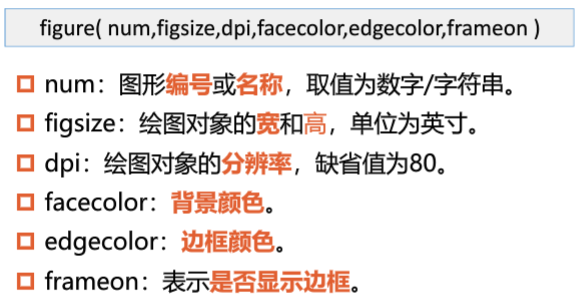1 2 3  pit.figure(figsize=(3,2),facecolor="green") #创建画布 plt.plot() #绘制空白图形 plt.show() #显示绘图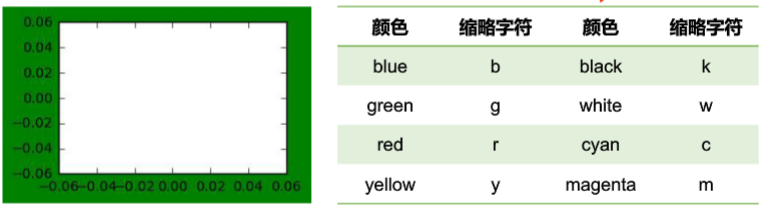subplot()函数——划分子图 subplot(行数,列数,子图序号) 例：将画布划分为2x2的子图区域，并绘制3个子图

 1 2 3 4 5  fig = plt.figure() plt.subplot(2,2,1) plt.subplot(2,2,2) plt.subplot(2,2,3) plt.show()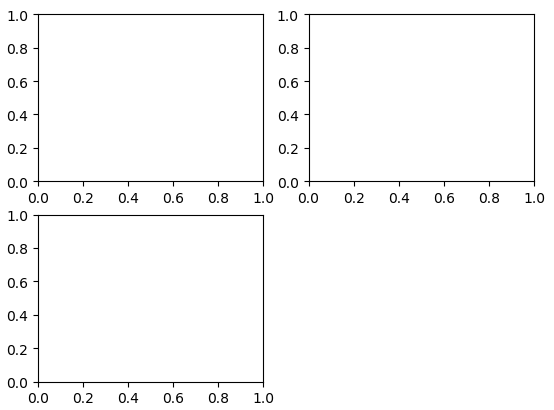plt.rcParams[]——设置中文字体 matplotlib库中的文字为英文，如果需要中文时容易乱码，例如：使用plt.rcParams[“font.sans-serif”] = “SimHei"使默认字体改为中文。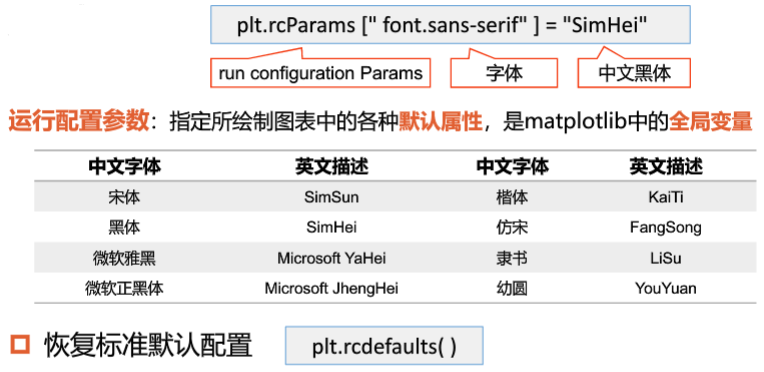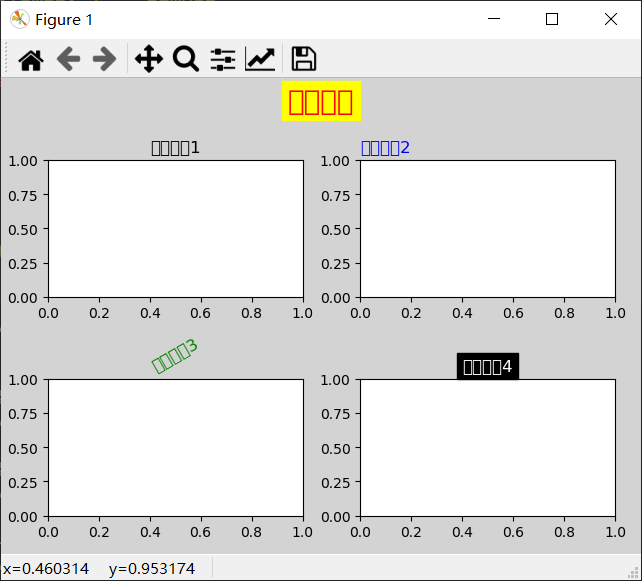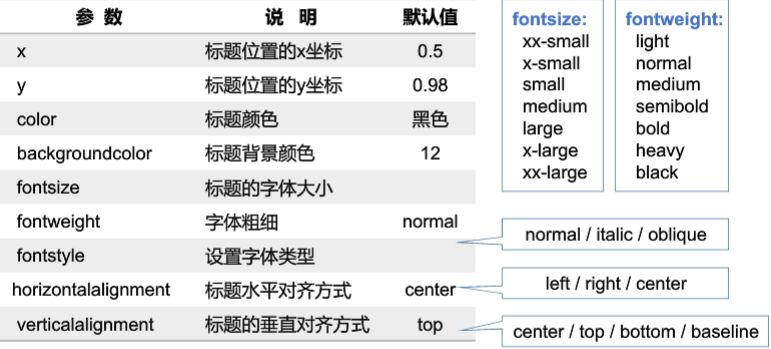title()函数的主要参数：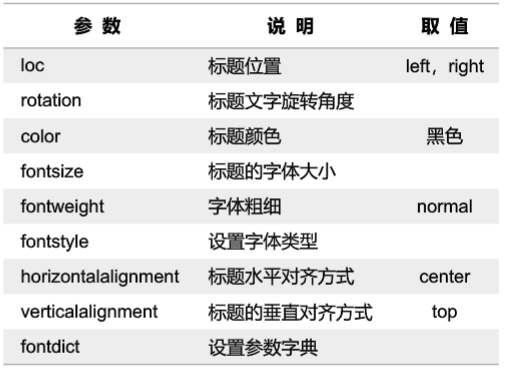tight_layout()函数 检查坐标轴标签、刻度标签和子图标题，自动调整子图，使之填充整个绘图区域，并消除子图之间的重叠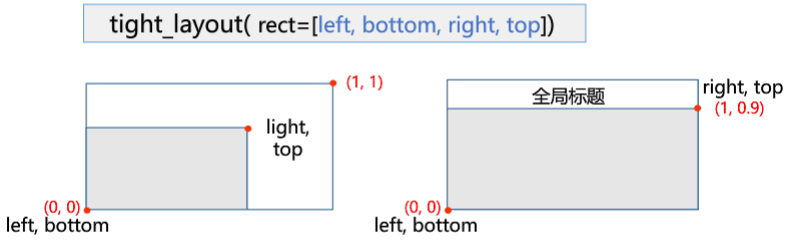SUMMARIZE:

  1 2 3 4 5 6 7 8 9 10 11 12 13 14 15 16 17  import matplotlib.pyplot as plt plt.rcParams["font.family"] = "SimHei" fig = plt.figure(facecolor = "lightgrey") plt.subplot(2,2,1) plt.title('子图标题1') plt.subplot(2,2,2) plt.title('子图标题2',loc="left",color="b") plt.subplot(2,2,3) myfontdict = {"fontsize":12,"color":"g","rotation":30} plt.title('子图标题3',fontdict=myfontdict) plt.subplot(2,2,4) plt.title('子图标题4',color = "white",backgroundcolor="black") plt.suptitle("全局标题",fontsize=20,color="red",backgroundcolor="yellow") plt.tight_layout(rect=[0,0,1,0.9]) plt.show()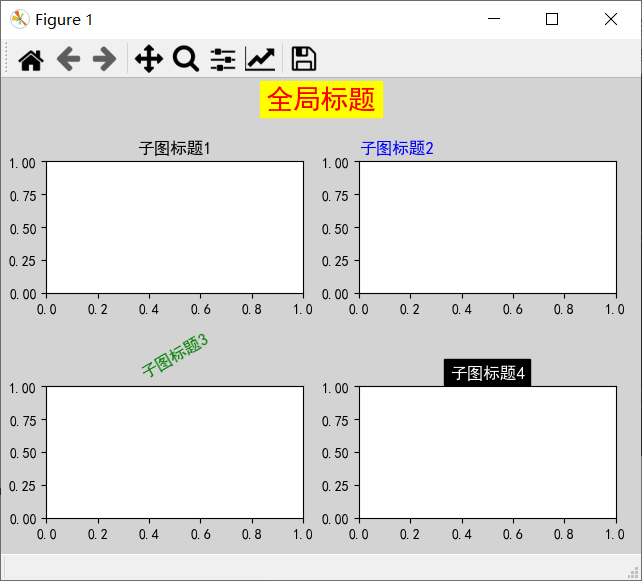### 2.散点图（Scatter）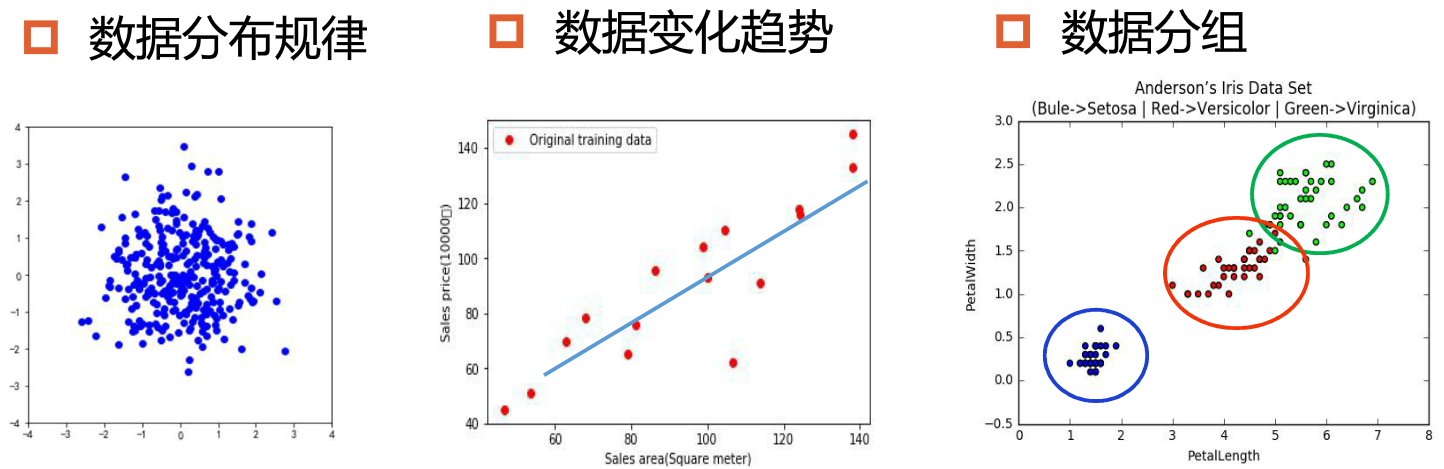scatter()函数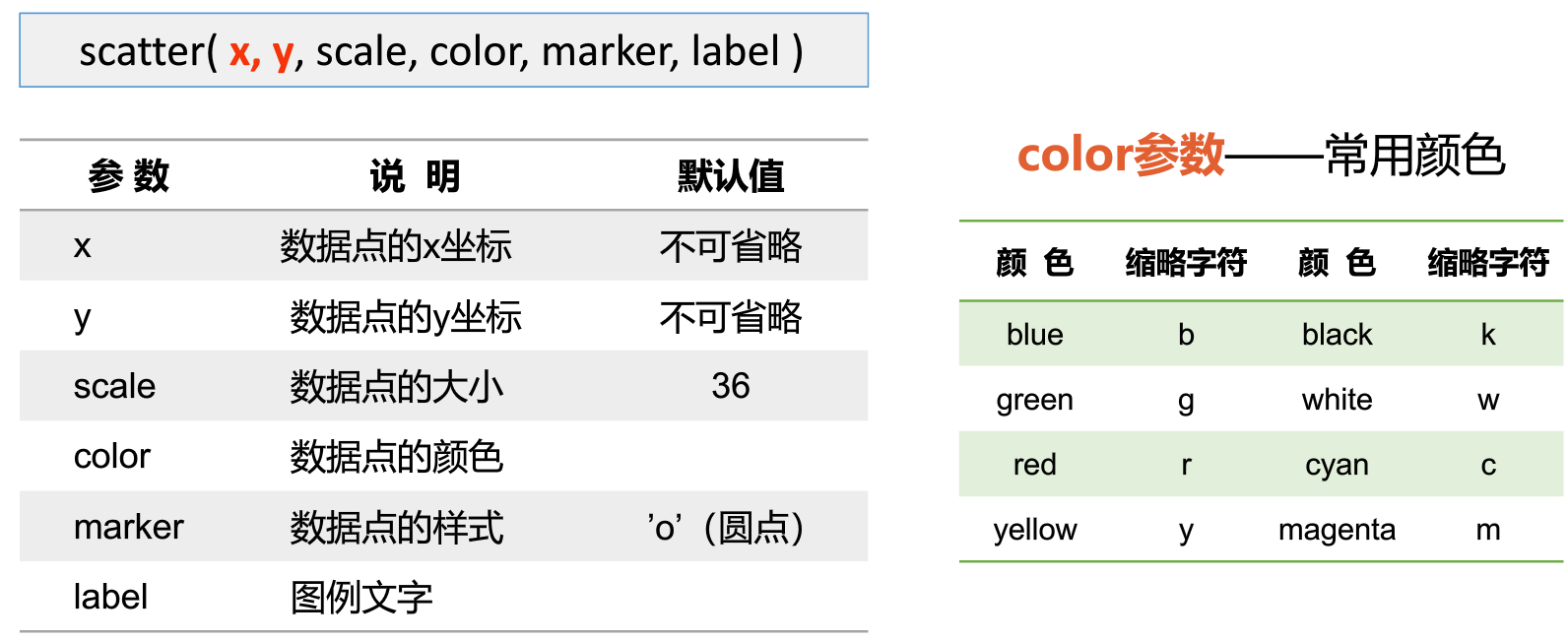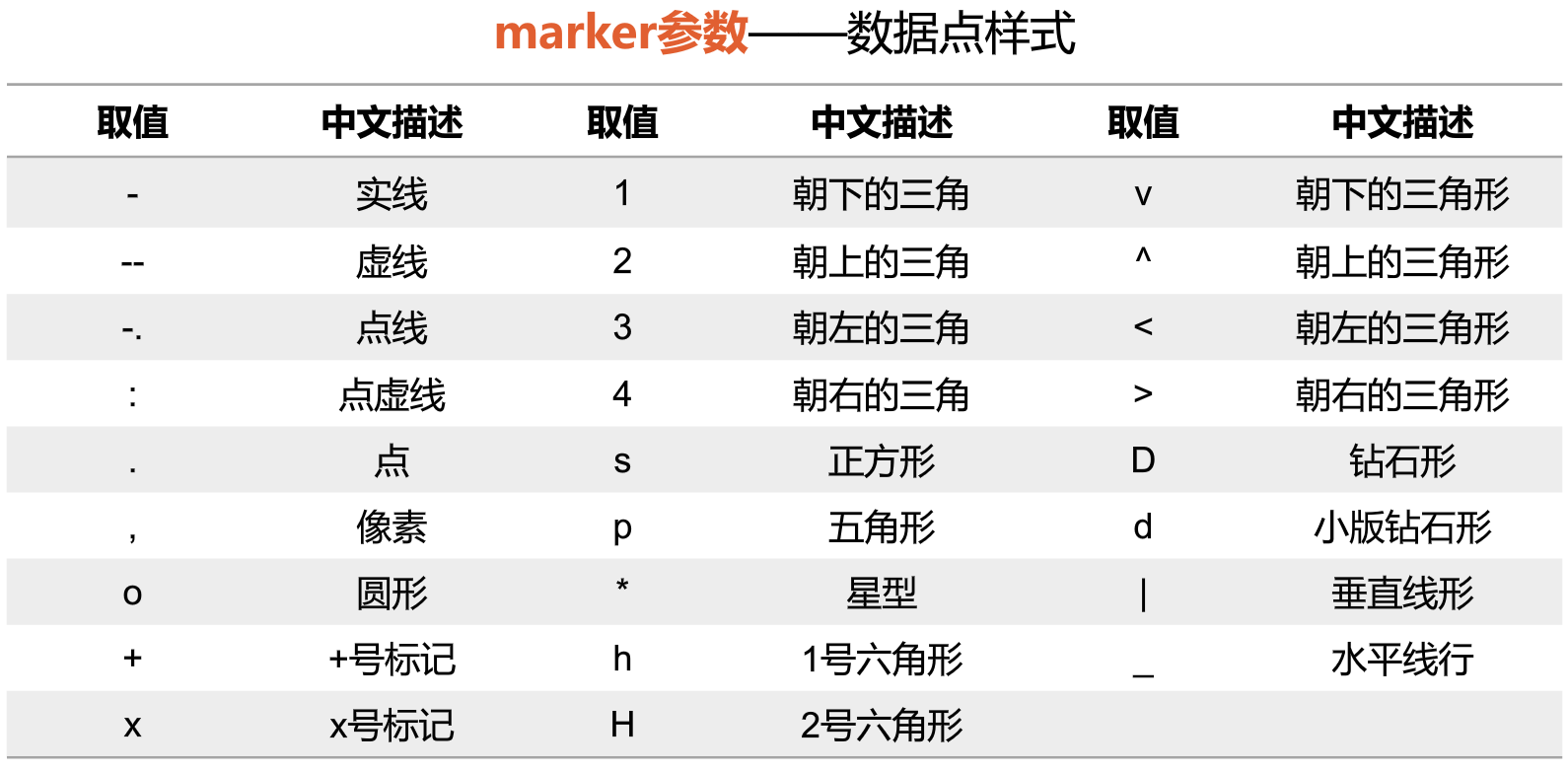text()函数 添加文字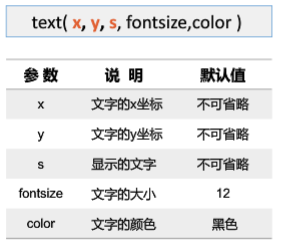xlabel(),ylabel()函数 设置坐标轴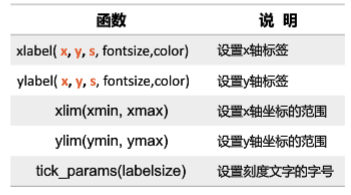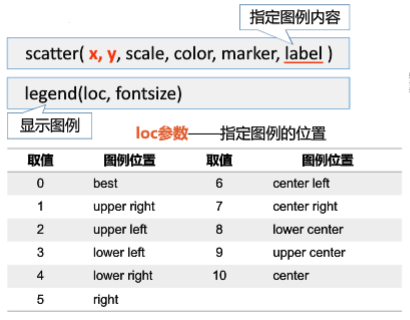1 2 3 4 5 6 7 8 9 10 11 12 13 14 15 16 17 18 19 20  import matplotlib.pyplot as plt import numpy as np plt.rcParams["font.sans-serif"]="SimHei" #设置中文字体为默认字体 plt.rcParams["axes.unicode_minus"]=False #使“-”正常显示 n = 1024 #随机点个数：1024 x = np.random.normal(0,1,n) #生成数据点x坐标 y = np.random.normal(0,1,n) #生成数据点y坐标 plt.scatter(x,y,color="blue",marker=".") #绘制数据点 plt.title("标准正态分布",fontsize=20) #设置标题 plt.text(2.5,2.5,"均 值：0\n标准差：1") #显示文本 plt.xlim(-4,4) #x轴范围 plt.ylim(-4,4) #y轴范围 plt.xlabel('横坐标x',fontsize=14) #x轴名称 plt.ylabel('纵坐标y',fontsize=14) #y轴名称 plt.show() #显示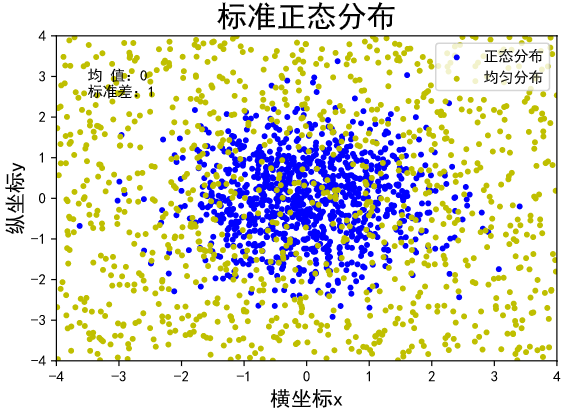### 3.折线图（Line Chart）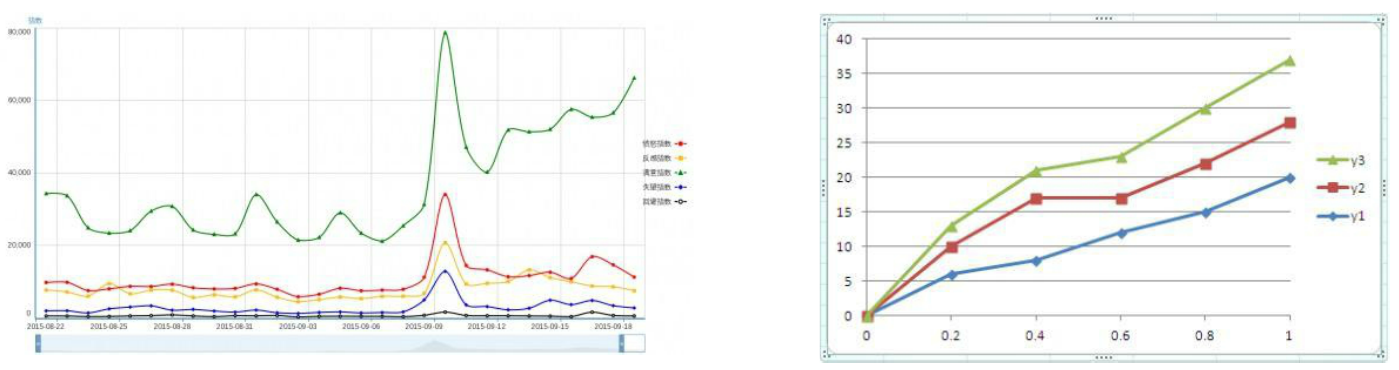plot()函数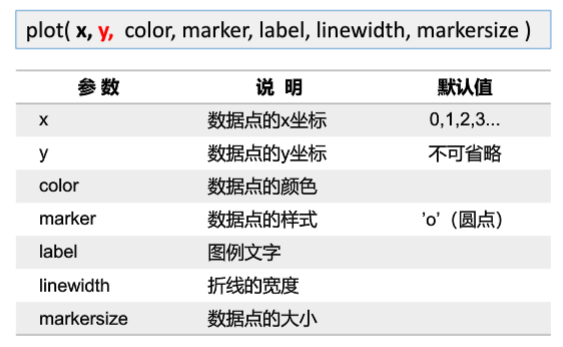1 2 3 4 5 6 7 8 9 10 11 12 13 14 15 16 17 18  import matplotlib.pyplot as plt import numpy as np plt.rcParams["font.sans-serif"]="SimHei" n = 24 y1 = np.random.randint(27,37,n) #生成随机数据 y2 = np.random.randint(40,60,n) plt.plot(y1, label="温度") #绘制 plt.plot(y2, label="湿度") plt.xlabel("小时",fontsize=12) plt.ylabel("测量值",fontsize=12) plt.title("24小时温度湿度统计",fontsize=16) plt.legend() plt.show()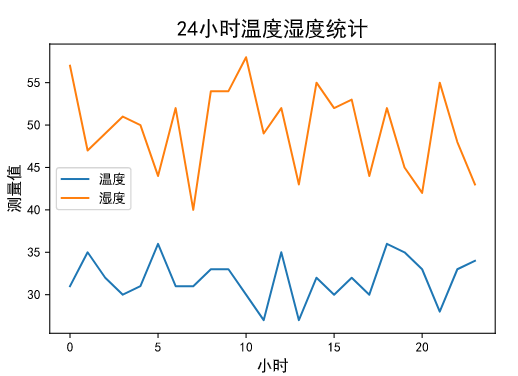### 4.柱形图（Bar Chart）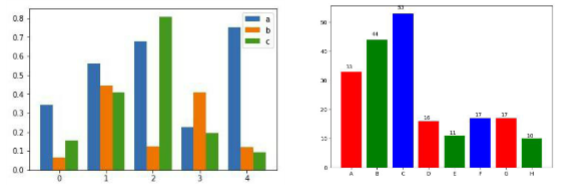bar()函数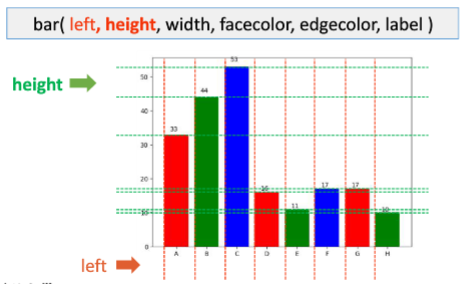1 2 3 4 5 6 7 8 9 10 11 12 13  import matplotlib.pyplot as plt import numpy as np plt.rcParams["font.sans-serif"]="SimHei" #设置中文字体为默认字体 plt.rcParams["axes.unicode_minus"]=False #使“-”正常显示 y1 = [32,25,32,35,45,33,17,24,20,10,32,5] y2 = [-14,-25,-18,-35,-46,-22,-18,-3,-24,-13,-25,-28] plt.bar(range(len(y1)),y1,width=0.8,facecolor="g",edgecolor="w",label="统计量1") plt.bar(range(len(y2)),y2,width=0.8,facecolor='r',edgecolor='w',label="统计量2") plt.title("柱状图",fontsize=20) plt.legend() plt.show()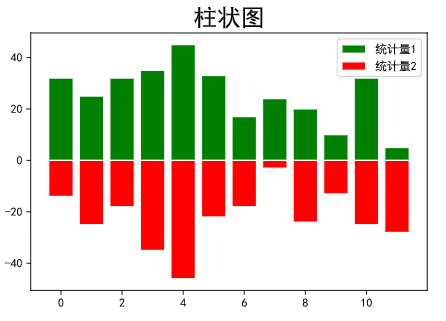### Matplotlib官网

http://matplotlib.org

https://matplotlib.org/genindex.html

Gallery页面